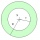# Body volume + annulus - math problems

#### Number of problems found: 5

• Round flowerbedAround a round flowerbed with a diameter of 6 meters and I will make a sidewalk up to 0.5 meters wide. How much gravel is needed if the layer is to be 5 cm high?
• Brass sphereFind the weight of a brass ball with an outer radius of 12 cm, a wall thickness of 20 mm if the density of the brass is 8.5 g/cm3.
• Metal tubeCalculate the metal tube mass 8dm long with the outer radius 5cm and the inner radius 4.5cm and 1cm3 of this metal is 9.5g.
• The coilHow many ropes (the diameter 8 mm) fit on the coil (threads are wrapped close together) The coil has dimension: the inner diameter 400mm, the outside diameter 800mm and the length of the coil is 470mm
• Steel tubeThe steel tube has an inner diameter of 4 cm and an outer diameter of 4.8 cm. The density of the steel is 7800 kg/m3. Calculate its length if it weighs 15 kg.

We apologize, but in this category are not a lot of examples.
Do you have an interesting mathematical word problem that you can't solve it? Submit a math problem, and we can try to solve it.

We will send a solution to your e-mail address. Solved examples are also published here. Please enter the e-mail correctly and check whether you don't have a full mailbox.

Please do not submit problems from current active competitions such as Mathematical Olympiad, correspondence seminars etc...

Body volume - math word problems. Annulus Problems.